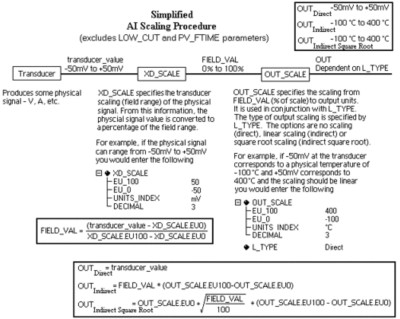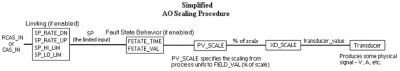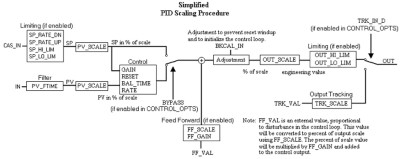# Introduction to FOUNDATION Fieldbus Scaling

## Overview

The scaling parameters are often confusing for new users. This document explains the basic scaling parameters and provides diagrams for the scaling of AI, AO, and PID blocks.

## Concept

The scaling parameters differ depending on the type of function block. For example, scaling on an AI will be different from scaling on an AO or PID block, although the basic concepts are the same.

For an Analog Input (AI) block, the most important scaling parameters are:
L_TYPE - The linearization type used in taking the physical transducer value to the block output.
XD_SCALE - Used in combination with L_TYPE to take values from the physical transducer for the I/O channel to a percent of scale. This percent of scale value is itself a parameter called FIELD_VAL.
OUT_SCALE - Used in combination with L_TYPE to take the percent of scale to an output value. This output value is called OUT.

The diagrams below will assist you in determining the parameters you need to change to obtain the correct scaling. Parameters relevant to scaling are listed in the last section.

## AI Diagram## AO Diagram## PID Diagram## Relevant Parameters

BYPASS
Allows the normal control algorithm in a PID block to be bypassed if the CONTROL_OPT parameter’s Bypass Enable option is selected. If control is bypassed, the PID uses its setpoint value (SP) in percent of scale as its output value and does not attempt to do any PID control. This parameter is used for secondary cascade PIDs that have a bad process variable (PV). BYPASS can only be changed when the block is in Man or OOS modes.

CONTROL_OPTS
A list of options used to adjust the way control blocks, such as the PID block, operate. The setting relevant to scaling is Bypass Enable. When set, it allows you to set the BYPASS parameter to bypass the algorithm’s control.

FF_GAIN
The gain by which the feed-forward input is multiplied before it is added to the output value of the control block.

FF_SCALE
The scaling parameter used by the feed-forward value of the block.

FF_VAL
The feed-forward value.

FIELD_VAL
The value from the input channel, in percent of scale.

FIELD_VAL_D
The value from the discrete input channel.

IN
The primary input of the block.

IN_1
The secondary input of the block.

IO_OPTS
A bitmask used to adjust the way I/O blocks (AI, DI, AO and DO) operate. The setting relevant to scaling is Low Cutoff. When set, it enables the AI low cutoff parameter.

L_TYPE
The linearization type. This parameter affects the way the value from the transducer is linearized in the analog input block before it is presented as the block output. In all cases, the FIELD_VAL parameter behaves as follows:

FIELD_VAL = (transducer_value - XD_SCALE.EU0) / (XD_SCALE.EU100 - XD_SCALE.EU0)

Linearization Types

 Type Description Direct The block output is directly taken from the transducer value: OUT = transducer_value Indirect The block output is scaled according to OUT_SCALE from the value in FIELD_VAL: OUT = OUT_SCALE.EU0 + ((FIELD_VAL / 100) * (OUT_SCALE.EU100 - OUT_SCALE.EU0)) Indirect Square Root The block output is scaled according to OUT_SCALE from the value in FIELD_VAL. Before the field value is rescaled, the square root is taken. OUT = OUT_SCALE.EU0 + SQRT(FIELD_VAL / 100) * (OUT_SCALE.EU100 - OUT_SCALE.EU0) Uninitialized An invalid setting. The device reports a configuration error with an Uninitalized L_TYPE.

LOW_CUT
With an L_TYPE of Indirect Square Root, this can be used to establish a floor (in percent of scale) for values from the transducer. If the calculated value is below this floor, it will be changed to zero. This is primarily useful for flow devices. This feature must first be enabled by setting the Low Cutoff option in the IO_OPTS parameter.

OUT
The current output value of the block.

OUT_SCALE
The scaling parameter used for the output parameter.

OUT_SCALE Parameter

 Subfield Meaning EU_100 Engineering units value at 100 percent of scale. EU_0 Engineering units value at zero percent of scale. UNIT_INDEX Actual engineering units code (such as mA). DECIMAL Number of digits a host shows to the right of the decimal for display purposes.

PV
The process variable being controlled by the process.

PV_FTIME
The filter time, in seconds, used in input blocks. For analog blocks, it is the time constant for a low pass exponential filter used to damp out rapid oscillations in the input value before using it as the process variable. For discrete blocks, it is the time the PV must remain constant after a change for the change to be reported.

PV_SCALE
The scaling parameter used by the process variable of the block. Converts from percent of scale to a process variable in engineering units. Contains the same subfields as OUT_SCALE.

READBACK
The valve or actuator position read back from the transducer, in transducer units.

SEL_1 through SEL_3
Input values for the selector.

SEL_TYPE
Defines the selector action - High, Medium, or Low.

TRK_IN_D
Used to enable tracking of the output value to TRK_VAL. When this is true, the output value of the block takes on the value specified in TRK_VAL.

TRK_SCALE
The scaling parameter used for the value specified by TRK_VAL.

TRK_VAL
The value the block will track when tracking is enabled by TRK_IN_D.

XD_SCALE
The scaling parameter used to interpret values from the physical I/O channel. This is used to translate from a physical transducer value to a percent of scale.

Scaling Parameter Values

 Subfield Meaning EU_100 Engineering units value at 100 percent of scale. EU_0 Engineering units value at zero percent of scale. UNIT_INDEX Actual engineering units code (such as mA). DECIMAL Number of digits a host shows to the right of the decimal for display purposes. (Not used by the NI-FBUS Configurator.)